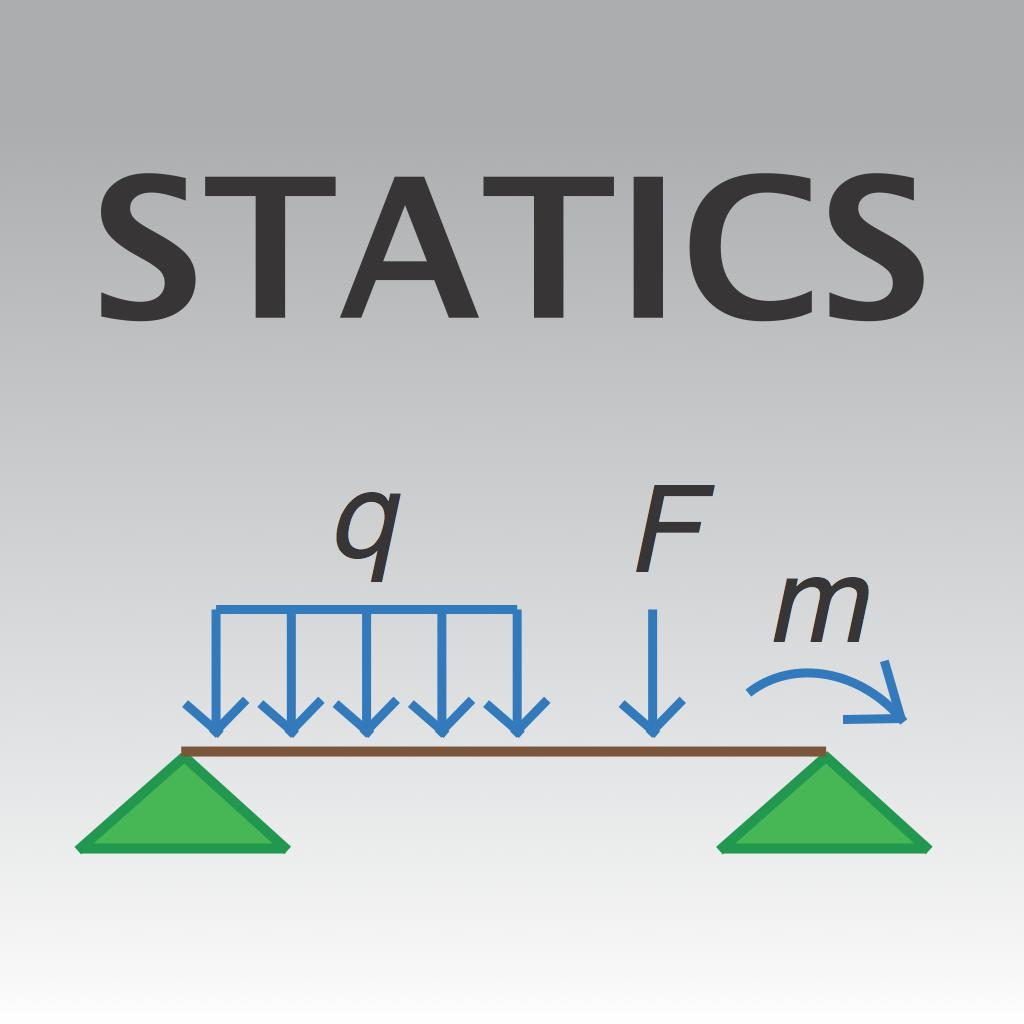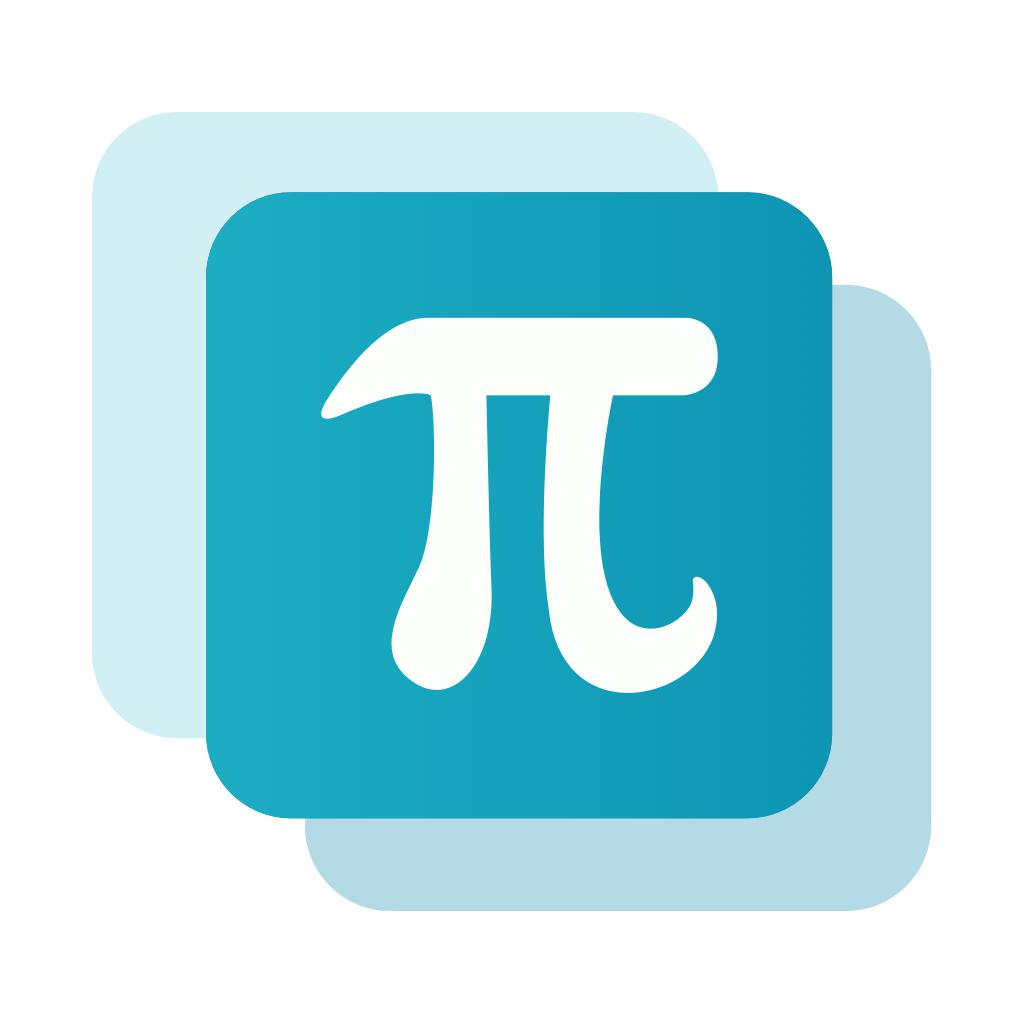"Mathway: Math Problem Solver" App Analytics: ASO Keyword Monitoring | ASOTools

# "Mathway: Math Problem Solver" App Analytics: ASO Keyword Monitoring | ASOTools

Are you doing Mathway: Math Problem Solver app analytics for better app store ASO strategies? Whether Mathway: Math Problem Solver in app store keep on top? What keyword tactics Mathway: Math Problem Solver take to rank well in app store? Are app store keywords like "mathway", "statics", "mathwa" matter in Mathway: Math Problem Solver ranking?

Free ASO tools for Google Play & App Store, ASOTools will reveal the app store keywords secrets of Mathway: Math Problem Solver.

## App Analytics: Mathway: Math Problem Solver

Mathway: Math Problem Solver-Homework scanner & calculator, published by Mathway, LLC in app store, released on 2012-09-04, and last updated 2021-05-06. It belongs to Education category, and Education category is ranking 3 in app store. Currently, Mathway: Math Problem Solver has 309194 ratings, the average rating is 5. Additionally, it had 700000 downloads and 1000000 revenue last month in app store worldwide, and the daily active users is -.

More Mathway: Math Problem Solver info and other app analytics can be found freely in ASOTool.

## ASO Keyword Monitoring: Mathway: Math Problem Solver

### 1.Mathway: Math Problem Solver Top 10 App Store Keywords

 Keyword Search Volume KD Related Apps Mathway: Math Problem Solver's Rank mathway 8 7856 29 31 1 statics 5 4605 53 219 1 mathwa 5 4605 73 231 1 math wat 11 4605 45 203 1 mathway free 35 4605 36 33 1 math wiz 5 4605 31 181 1 mathways 22 4605 47 33 1 mymath 5 4605 37 193 1 mathwah 5 4605 43 32 1 mathwau 11 4605 46 32 1

App store keywords matter for ASO. If your app is similar to Mathway: Math Problem Solver, when you optimize app store keyword ranking, pay attention to the metrics above. ASOTools will show you all of them for free.

Obviously, Mathway: Math Problem Solver is ranking for "mathway", "statics", "mathwa", "math wat", "mathway free", "math wiz", "mathways", "mymath", "mathwah", and "mathwau" these top 10 keywords in app store.

Also, you'll find Mathway: Math Problem Solver's position in app store search result. Mathway: Math Problem Solver is ranking 1 for mathway, ranking 1 for statics, ranking 1 for mathwa, and ranking 1 for math wat. For more Mathway: Math Problem Solver keyword monitoring, please visit asotools.io.

### 2. Top 5 Keyword-Related Apps& App Analytics

• mathway  Related Apps & App Analytics
 Apps Average Star RatingsMathway: Math Problem Solver-Homework scanner & calculator 5 309194Photomath-Math explained, step-by-step 5 456568MathPapa - Algebra Calculator-Algebra Calculator & Solver 4 13019FastMath - Take Photo & Solve-Math Problem Solver 4 29777Microsoft Math Solver - HW app-Math Homework Scanner & Tutor 5 20565

"mathway" has 31 apps. Top 5 are Mathway: Math Problem Solver, Photomath, MathPapa - Algebra Calculator, FastMath - Take Photo & Solve, and Microsoft Math Solver - HW app.

• statics  Related Apps & App Analytics
 Apps Average Star RatingsMathway: Math Problem Solver-Homework scanner & calculator 5 309194Photomath-Math explained, step-by-step 5 456568Statics (Civil Engineering) 2 4Symbolab Calculator-Math Solver with Steps 4 7814IXL - Math, English, & More-K-12 learning on the go 4 83251

"statics" has 219 related apps in total. Top 5 are Mathway: Math Problem Solver, Photomath, Statics (Civil Engineering), Symbolab Calculator, and IXL - Math, English, & More.

• mathwa  Related Apps & App Analytics
 Apps Average Star RatingsMathway: Math Problem Solver-Homework scanner & calculator 5 309194Photomath-Math explained, step-by-step 5 456568MathPapa - Algebra Calculator-Algebra Calculator & Solver 4 13019FastMath - Take Photo & Solve-Math Problem Solver 4 29777Socratic by Google-Get unstuck. Learn better. 5 353942

"mathwa" has 231 related apps in app store. Top 5 are Mathway: Math Problem Solver, Photomath, MathPapa - Algebra Calculator, FastMath - Take Photo & Solve, and Socratic by Google.

• math wat  Related Apps & App Analytics
 Apps Average Star RatingsMathway: Math Problem Solver-Homework scanner & calculator 5 309194Photomath-Math explained, step-by-step 5 456568FastMath - Take Photo & Solve-Math Problem Solver 4 29777MathPapa - Algebra Calculator-Algebra Calculator & Solver 4 13019Brainly – Homework Help App-Homework App, Homework Answers 3 13634

"math wat" has 203 related apps. Top 5 apps are Mathway: Math Problem Solver, Photomath, FastMath - Take Photo & Solve, MathPapa - Algebra Calculator, and Brainly – Homework Help App.

• mathway free  Related Apps & App Analytics
 Apps Average Star RatingsMathway: Math Problem Solver-Homework scanner & calculator 5 309194Photomath-Math explained, step-by-step 5 456568Cymath - Math Problem Solver-Math Homework Help 4 9498Microsoft Math Solver - HW app-Math Homework Scanner & Tutor 5 20565MathMe - Problem Solver-Math Problems, Step-By-Step 4 2525

33 apps are ranking for "mathway free" in app store. Top 5 apps are Mathway: Math Problem Solver, Photomath, Cymath - Math Problem Solver, Microsoft Math Solver - HW app, and MathMe - Problem Solver.

As you can see, Mathway: Math Problem Solver, Mathway: Math Problem Solver, and Photomath are ranking for mathway, also, Mathway: Math Problem Solver, Mathway: Math Problem Solver, Photomath and Symbolab Calculator are all competing the keyword statics.

Before promoting your apps in app store, you'd better perform app analytics for better ASO tactics, and ASOTools, free ASO tools will help you with App store keywords optimization.